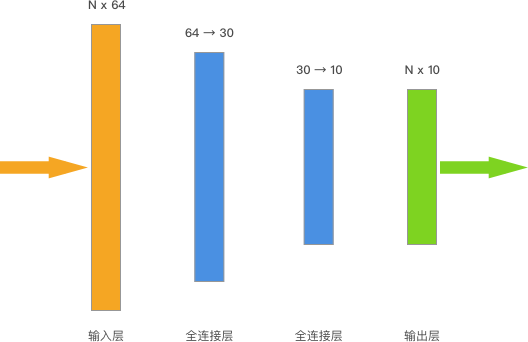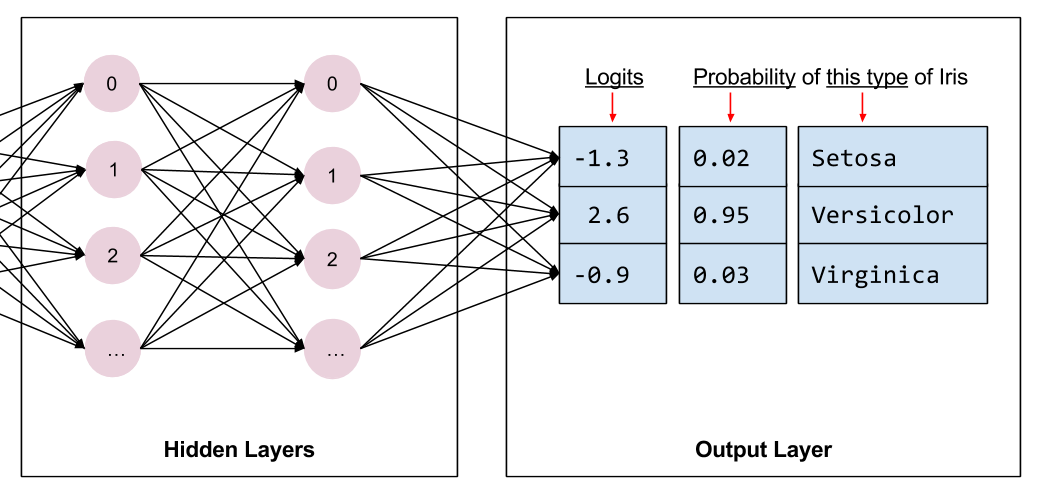## 深度学习：TensorFlow 构建神经网络

6940 字 · 162 阅读 · 2023 年 05 月 24 日### 介绍

TensorFlow 作为深度学习框架，当然是为了帮助我们更便捷地构建神经网络。所以，这篇文章将会了解如何使用 TensorFlow 来构建神经网络，并学会 TensorFlow 构建神经网络的重要函数和方法。

### 知识点

• NumPy 构建神经网络
• TensorFlow 构建神经网络
• TensorFlow 完成 DIGITS 分类
• TensorFlow 实现 Mini Batch 训练

### NumPy 构建神经网络import numpy as np

class NeuralNetwork:
def __init__(self, X, y, lr):
"""初始化参数"""
self.input_layer = X
self.W1 = np.ones((self.input_layer.shape, 3))  # 初始化权重全为 1
self.W2 = np.ones((3, 1))
self.y = y
self.lr = lr

def forward(self):
"""前向传播"""
self.hidden_layer = sigmoid(np.dot(self.input_layer, self.W1))
self.output_layer = sigmoid(np.dot(self.hidden_layer, self.W2))
return self.output_layer

def backward(self):
"""反向传播"""
d_W2 = np.dot(self.hidden_layer.T, (2 * (self.output_layer - self.y) *
sigmoid_derivative(np.dot(self.hidden_layer, self.W2))))

d_W1 = np.dot(self.input_layer.T, (
np.dot(2 * (self.output_layer - self.y) * sigmoid_derivative(
np.dot(self.hidden_layer, self.W2)), self.W2.T) * sigmoid_derivative(
np.dot(self.input_layer, self.W1))))

# 参数更新
self.W1 -= self.lr * d_W1
self.W2 -= self.lr * d_W2


import pandas as pd

# 直接运行加载数据集

from matplotlib import pyplot as plt
%matplotlib inline

X = df[['X0', 'X1']].values  # 输入值
y = df[['Y']].values  # 真实 y
nn_model = NeuralNetwork(X, y, lr=0.001)  # 定义模型
loss_list = []  # 存放损失数值变化

def sigmoid(x):
"""激活函数"""
return 1 / (1 + np.exp(-x))

def sigmoid_derivative(x):
"""sigmoid 函数求导"""
return sigmoid(x) * (1 - sigmoid(x))

# 迭代 200 次
for _ in range(200):
y_ = nn_model.forward()  # 前向传播
nn_model.backward()  # 反向传播
loss = np.square(np.subtract(y, y_)).mean()  # 计算 MSE 损失
loss_list.append(loss)

plt.plot(loss_list)  # 绘制 loss 曲线变化图
plt.title(f"final loss: {loss}")


### TensorFlow 构建神经网络

#### 处理张量数据

import tensorflow as tf

# 将数组转换为常量张量
X = tf.cast(tf.constant(df[['X0', 'X1']].values), tf.float32)
y = tf.constant(df[['Y']].values)

X.shape, y.shape


#### 定义模型类

class Model(object):
def __init__(self):
# 初始化权重全为 1，也可以随机初始化
# 选择变量张量，因为权重后续会不断迭代更新
self.W1 = tf.Variable(tf.ones([2, 3]))
self.W2 = tf.Variable(tf.ones([3, 1]))

def __call__(self, x):
hidden_layer = tf.nn.sigmoid(tf.linalg.matmul(X, self.W1))  # 隐含层前向传播
y_ = tf.nn.sigmoid(tf.linalg.matmul(hidden_layer, self.W2))  # 输出层前向传播
return y_


model = Model()  # 实例化类
y_ = model(X)  # 测试输入
y_.shape  # 输出


#### MSE 损失函数

def loss_fn(model, X, y):
y_ = model(X)  # 前向传播得到预测值
# 使用 MSE 损失函数，并使用 reduce_mean 计算样本总损失
loss = tf.reduce_mean(tf.losses.mean_squared_error(y_true=y, y_pred=y_))
return loss


loss = loss_fn(model, X, y)
loss


#### 梯度下降优化迭代

EPOCHS = 200  # 迭代 200 次
LEARNING_RATE = 0.1  # 学习率

for epoch in range(EPOCHS):
loss = loss_fn(model, X, y)  # 计算 Loss，包含前向传播过程
# 使用梯度下降法优化迭代
# 输出模型需优化参数 W1，W2 自动微分结果
dW1, dW2 = tape.gradient(loss, [model.W1, model.W2])
model.W1.assign_sub(LEARNING_RATE * dW1)  # 更新梯度
model.W2.assign_sub(LEARNING_RATE * dW2)

# 每 100 个 Epoch 输出各项指标
if epoch == 0:
print(f'Epoch [000/{EPOCHS}], Loss: [{loss:.4f}]')
elif (epoch + 1) % 100 == 0:
print(f'Epoch [{epoch+1}/{EPOCHS}], Loss: [{loss:.4f}]')


#### 使用 TensorFlow 优化器

TensorFlow 优化器使用非常方便。如下所示，定义优化器并设定学习率。

# 定义 SGD 优化器，设定学习率，
optimizer = tf.optimizers.SGD(learning_rate=0.1)
optimizer


loss_list = []  # 存放每一次 loss
model = Model()  # 实例化类
for epoch in range(EPOCHS):
loss = loss_fn(model, X, y)  # 计算 Loss，包含前向传播过程
loss_list.append(loss)  # 保存每次迭代 loss

# 每 100 个 Epoch 输出各项指标
if epoch == 0:
print(f'Epoch [000/{EPOCHS}], Loss: [{loss:.4f}]')
elif (epoch + 1) % 100 == 0:
print(f'Epoch [{epoch+1}/{EPOCHS}], Loss: [{loss:.4f}]')


plt.plot(loss_list)  # 绘制 loss 变化图像


class Model(object):
def __init__(self):
self.W1 = tf.Variable(tf.ones([2, 3]))
self.W2 = tf.Variable(tf.ones([3, 1]))

def __call__(self, x):
hidden_layer = tf.nn.sigmoid(tf.linalg.matmul(X, self.W1))
y_ = tf.nn.sigmoid(tf.linalg.matmul(hidden_layer, self.W2))
return y_

def loss_fn(model, X, y):
y_ = model(X)
loss = tf.reduce_mean(tf.losses.mean_squared_error(y_true=y, y_pred=y_))
return loss

X = tf.cast(tf.constant(df[['X0', 'X1']].values), tf.float32)
y = tf.constant(df[['Y']].values)

model = Model()
EPOCHS = 200

for epoch in range(EPOCHS):
loss = loss_fn(model, X, y)
optimizer = tf.optimizers.SGD(learning_rate=0.1)


• NumPy 构建神经网络：定义数据 → 前向传播 → 手动推导梯度计算公式 → 反向传播 → 更新权重 → 迭代优化。
• TensorFlow 构建神经网络：定义张量 → 定义前向传播模型 → 定义损失函数 → 定义优化器 → 迭代优化。

### DIGITS 分类

DIGITS 手写字符数据集是机器学习中基础而经典的问题了，TensorFlow 构建神经网络的例子中，我们同样使用这个简单的数据集来练习。数据集加载和切分过程中，我们使用 scikit-learn 提供的 API 来完成。

from sklearn.datasets import load_digits

digits_X = digits.data  # 特征值
digits_y = digits.target  # 标签值

digits_X.shape, digits_y.shape


#### 数据预处理

0 1 0 0 0 0 0 0 0 0 0
1 0 1 0 0 0 0 0 0 0 0
2 0 0 1 0 0 0 0 0 0 0
3 0 0 0 1 0 0 0 0 0 0
4 0 0 0 0 1 0 0 0 0 0
5 0 0 0 0 0 1 0 0 0 0
6 0 0 0 0 0 0 1 0 0 0
7 0 0 0 0 0 0 0 1 0 0
8 0 0 0 0 0 0 0 0 1 0
9 0 0 0 0 0 0 0 0 0 1

digits_y = np.eye(10)[digits_y.reshape(-1)]
digits_y


from sklearn.model_selection import train_test_split

X_train, X_test, y_train, y_test = train_test_split(
digits_X, digits_y, test_size=0.2, random_state=1)

X_train.shape, X_test.shape, y_train.shape, y_test.shape


#### 定义模型类import tensorflow as tf

class Model(object):
def __init__(self):
# 随机初始化张量参数
self.W1 = tf.Variable(tf.random.normal([64, 30]))
self.b1 = tf.Variable(tf.random.normal())
self.W2 = tf.Variable(tf.random.normal([30, 10]))
self.b2 = tf.Variable(tf.random.normal())

def __call__(self, x):
x = tf.cast(x, tf.float32)  # 转换输入数据类型
# 线性计算 + RELU 激活
fc1 = tf.nn.relu(tf.add(tf.matmul(x, self.W1), self.b1))  # 全连接层 1
fc2 = tf.add(tf.matmul(fc1, self.W2), self.b2)  # 全连接层 2
return fc2


#### 交叉熵损失函数

$$H_{y^{\prime}}(y)=-\sum_{i} y_{i}^{\prime} \log \left(y_{i}\right)$$

$$\operatorname{softmax}(x)_{i}=\frac{\exp \left(x_{i}\right)}{\sum_{j} \exp \left(x_{j}\right)}$$def softmax(x):
# Softmax 实现
exp_x = np.exp(x)
return exp_x / np.sum(exp_x)

np.round(softmax([-1.3, 2.6, -0.9]), 2)


def loss_fn(model, x, y):
preds = model(x)
return tf.reduce_mean(tf.nn.softmax_cross_entropy_with_logits(logits=preds, labels=y))


EPOCHS = 200  # 迭代此时
LEARNING_RATE = 0.02  # 学习率
model = Model()  # 实例化模型类
for epoch in range(EPOCHS):
with tf.GradientTape() as tape:  # 追踪梯度
loss = loss_fn(model, X_train, y_train)

trainable_variables = [model.W1, model.b1, model.W2, model.b2]  # 需优化参数列表

# 每 100 个 Epoch 输出各项指标
if epoch == 0:
print(f'Epoch [000/{EPOCHS}], Loss: [{loss:.4f}]')
elif (epoch + 1) % 100 == 0:
print(f'Epoch [{epoch+1}/{EPOCHS}], Loss: [{loss:.4f}]')


def accuracy_fn(logits, labels):
preds = tf.argmax(logits, axis=1)  # 取值最大的索引，正好对应字符标签
labels = tf.argmax(labels, axis=1)
return tf.reduce_mean(tf.cast(tf.equal(preds, labels), tf.float32))


EPOCHS = 500  # 迭代此时
LEARNING_RATE = 0.02  # 学习率
model = Model()  # 实例化模型类
for epoch in range(EPOCHS):
with tf.GradientTape() as tape:  # 追踪梯度
loss = loss_fn(model, X_train, y_train)

trainable_variables = [model.W1, model.b1, model.W2, model.b2]  # 需优化参数列表

accuracy = accuracy_fn(model(X_test), y_test)  # 计算准确度

# 每 100 个 Epoch 输出各项指标
if epoch == 0:
print(f'Epoch [000/{EPOCHS}], Accuracy: [{accuracy:.2f}], Loss: [{loss:.4f}]')
elif (epoch + 1) % 100 == 0:
print(f'Epoch [{epoch+1}/{EPOCHS}], Accuracy: [{accuracy:.2f}], Loss: [{loss:.4f}]')


### TensorFlow 实现 Mini Batch 训练

from sklearn.model_selection import KFold
from tqdm.notebook import tqdm

EPOCHS = 500  # 迭代此时
BATCH_SIZE = 64  # 每次迭代的批量大小
LEARNING_RATE = 0.02  # 学习率

model = Model()  # 实例化模型类

for epoch in tqdm(range(EPOCHS)):  # 设定全数据集迭代次数
indices = np.arange(len(X_train))  # 生成训练数据长度规则序列
np.random.shuffle(indices)  # 对索引序列进行打乱，保证为随机数据划分
batch_num = int(len(X_train)/BATCH_SIZE)  # 根据批量大小求得要划分的 batch 数量
kf = KFold(n_splits=batch_num)  # 将数据分割成 batch 数量份
# KFold 划分打乱后的索引序列，然后依据序列序列从数据中抽取 batch 样本
for _, index in kf.split(indices):
X_batch = X_train[indices[index]]  # 按打乱后的序列取出数据
y_batch = y_train[indices[index]]

with tf.GradientTape() as tape:  # 追踪梯度
loss = loss_fn(model, X_batch, y_batch)

trainable_variables = [model.W1, model.b1,
model.W2, model.b2]  # 需优化参数列表

accuracy = accuracy_fn(model(X_test), y_test)  # 计算准确度

# 每 100 个 Epoch 输出各项指标
if epoch == 0:
print(f'Epoch [000/{EPOCHS}], Accuracy: [{accuracy:.2f}], Loss: [{loss:.4f}]')
elif (epoch + 1) % 100 == 0:
print(f'Epoch [{epoch+1}/{EPOCHS}], Accuracy: [{accuracy:.2f}], Loss: [{loss:.4f}]')


#### 小贴士### 系列文章•